# Selina Solutions Concise Maths Class 7 Chapter 9: Profit, Loss and Discount

Selina Solutions Concise Maths Class 7 Chapter 9 Profit, Loss and Discount provides the basic idea about the concepts, which are important from the exam point of view. The various examples present in the textbook help students understand the method of determining the cost price, selling price and profit/ loss. Students can use Selina Solutions Concise Maths Class 7 Chapter 9 Profit, Loss and Discount PDF, from the links available below.

Chapter 9 has problems in finding the C.P, S.P, Profit percent and Loss percent in a simple manner. The interactive step wise explanations provided in the solutions help students gain a hold on the concepts.

## Selina Solutions Concise Maths Class 7 Chapter 9: Profit, Loss and Discount Download PDF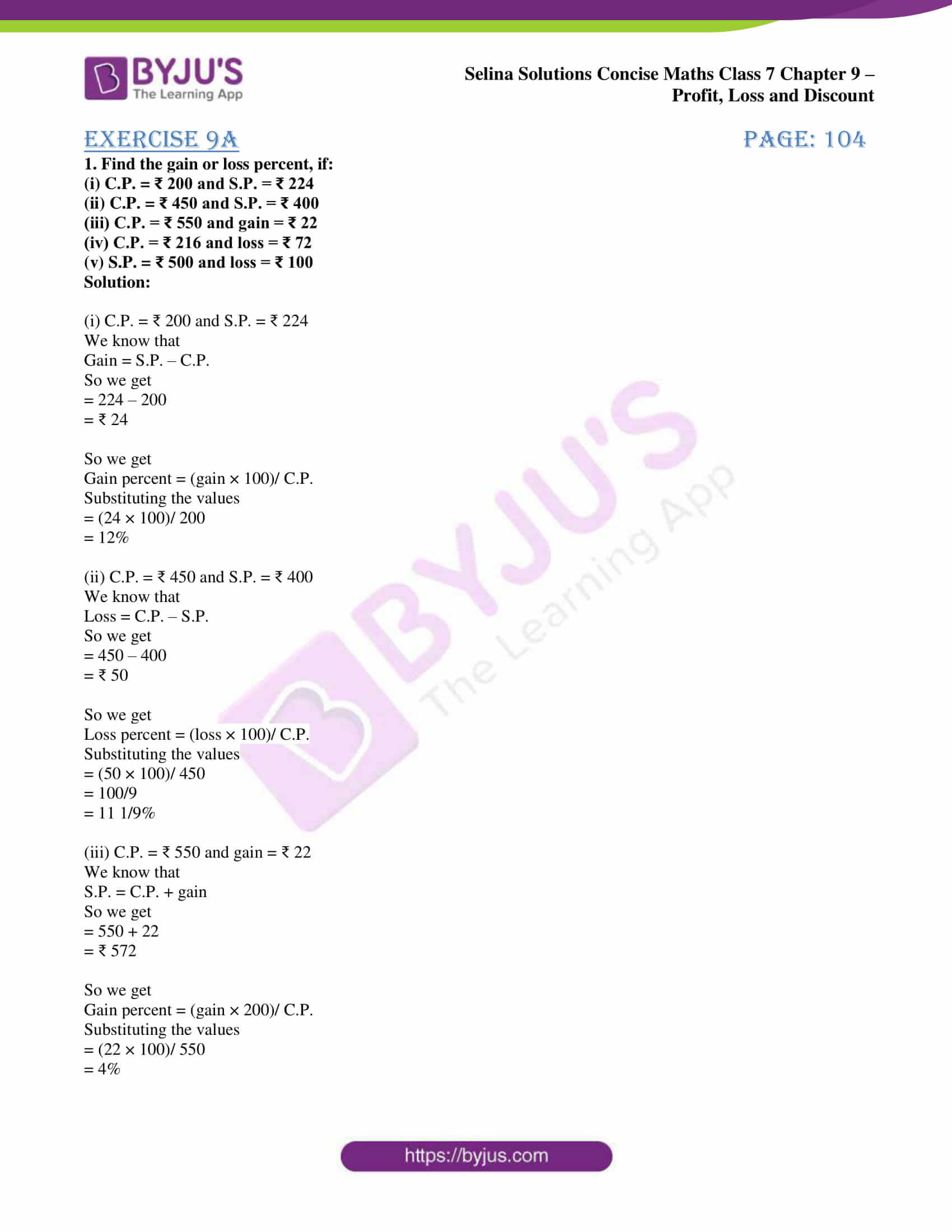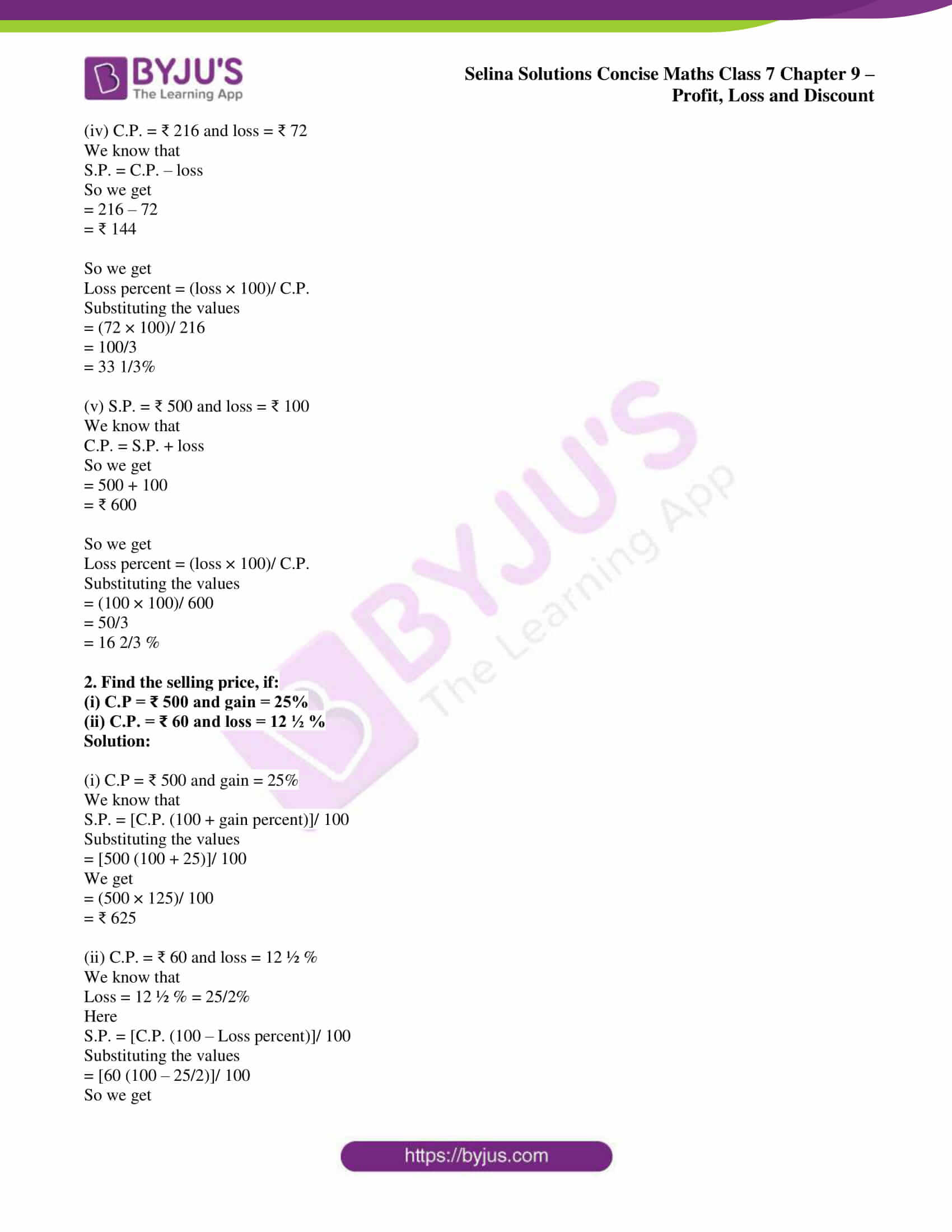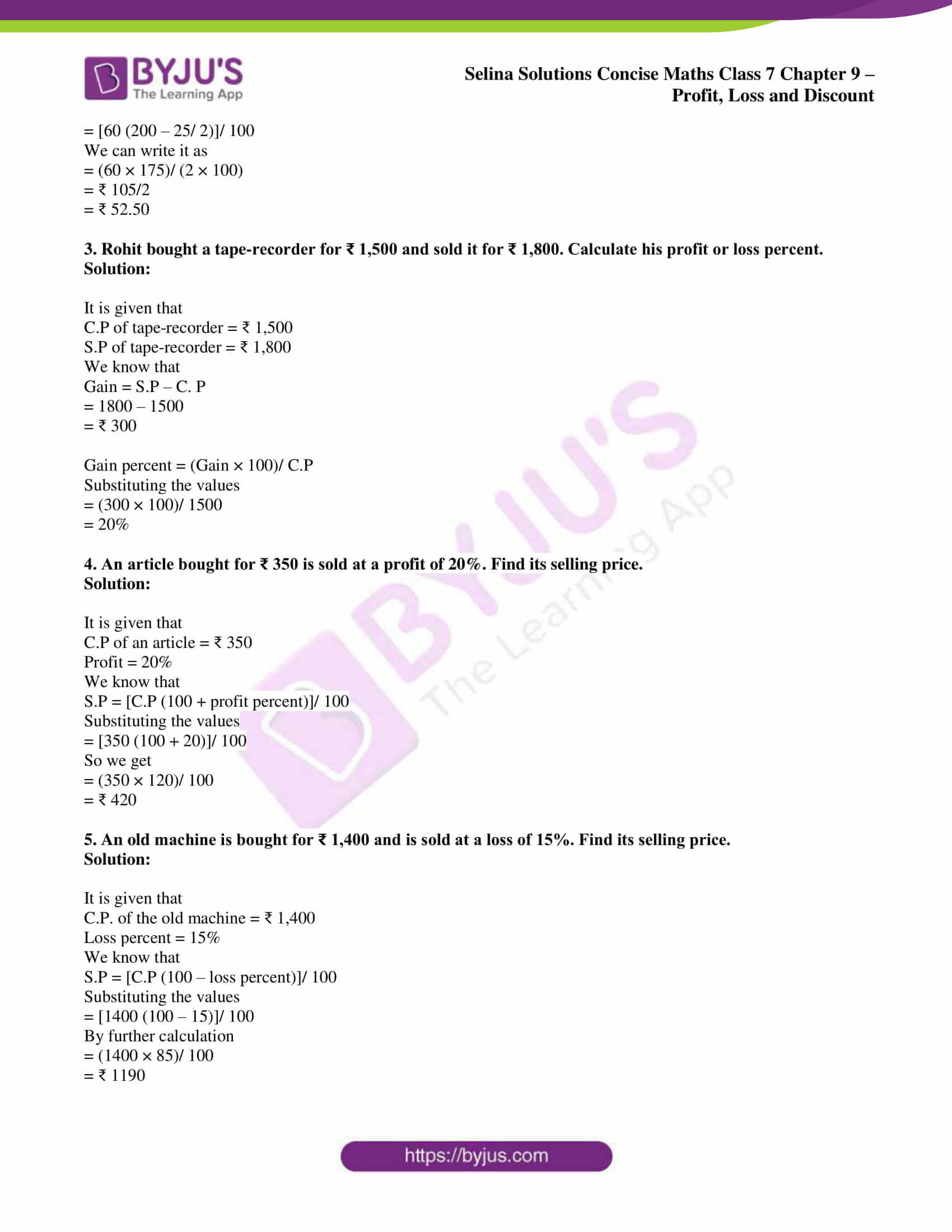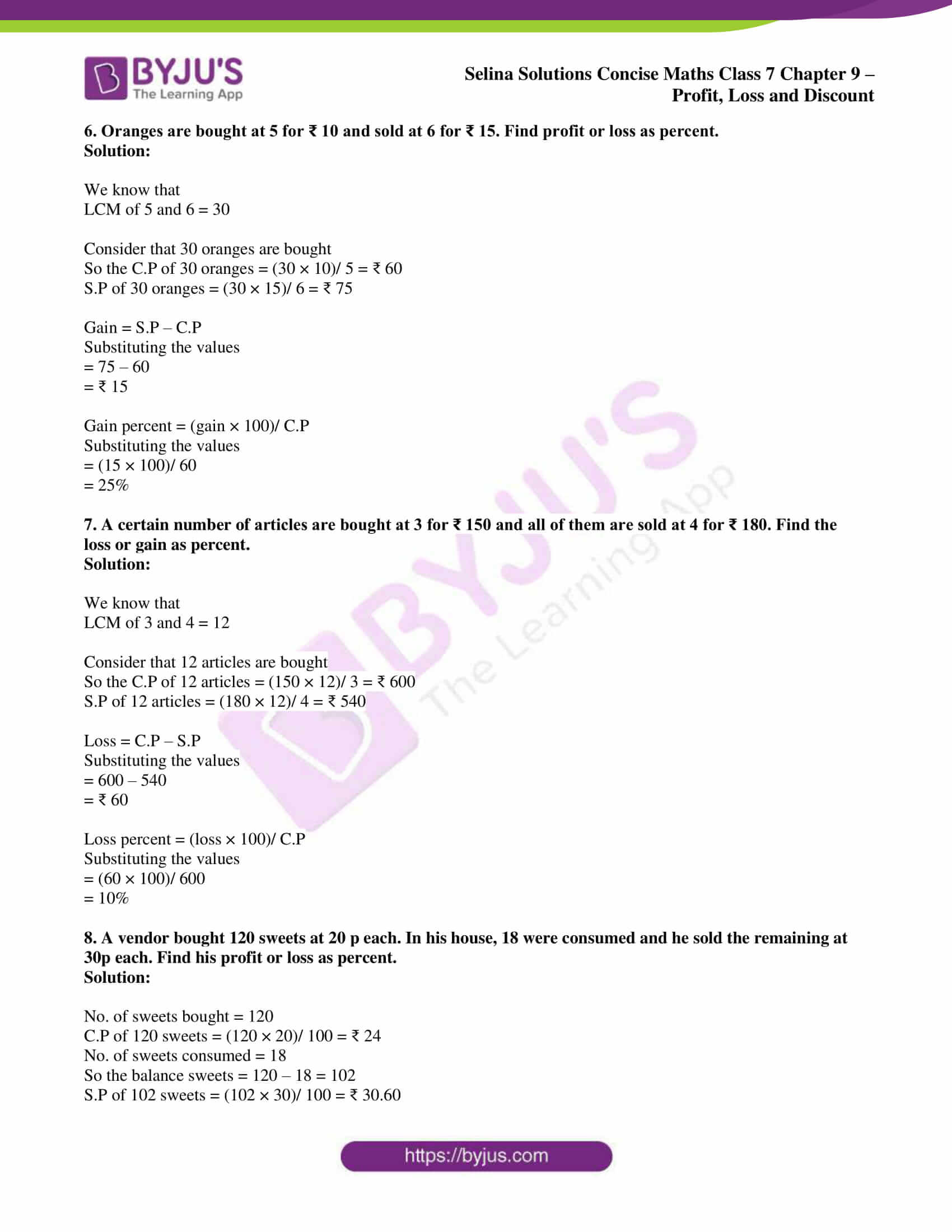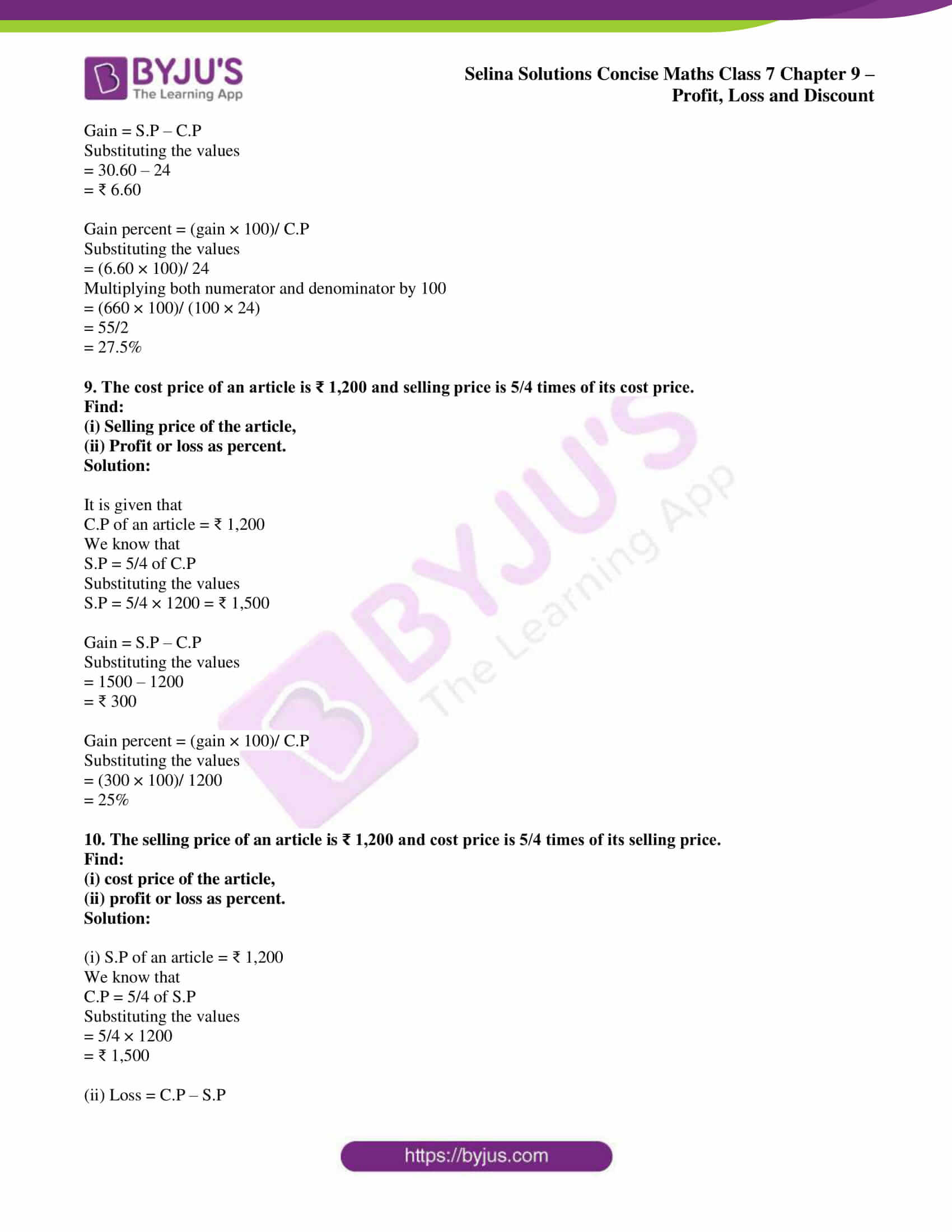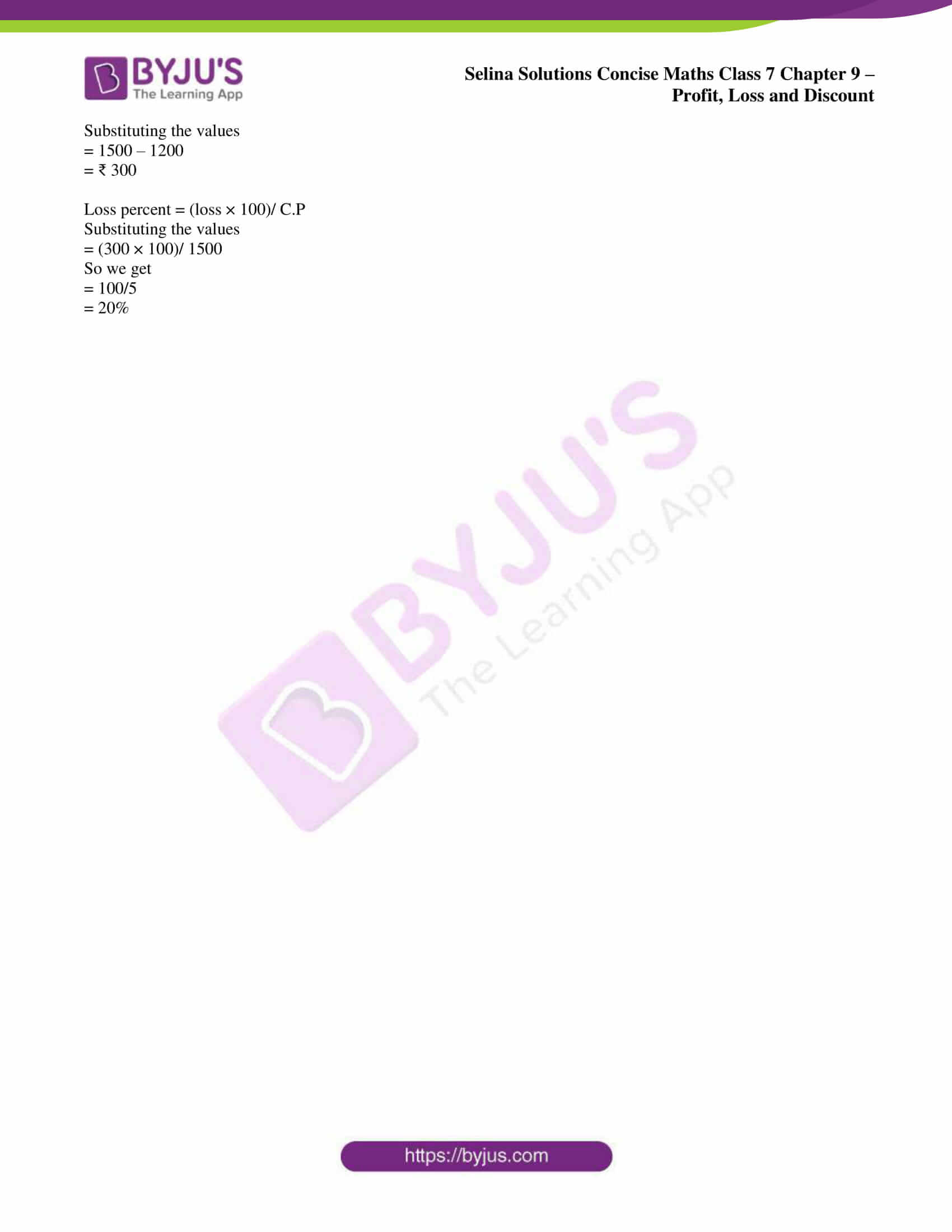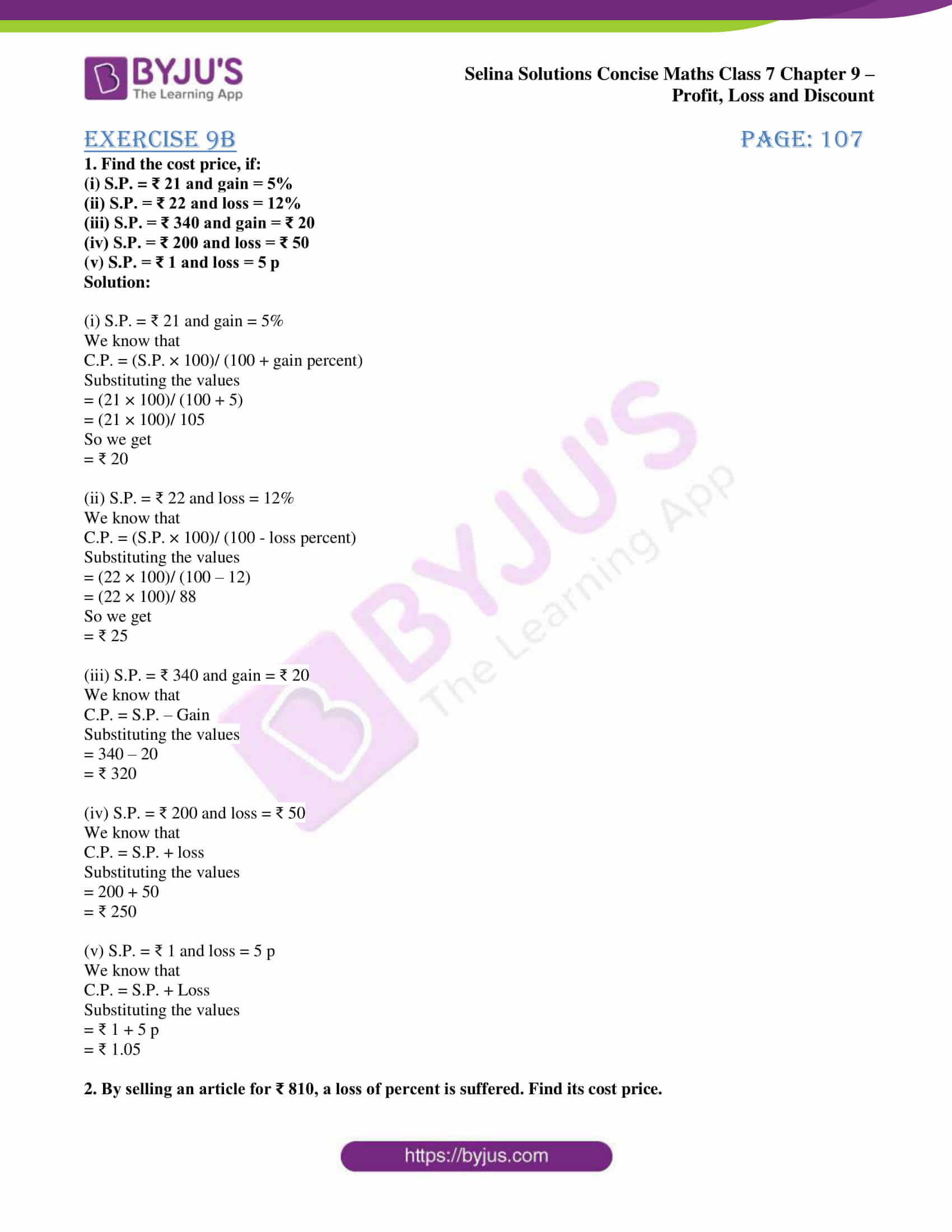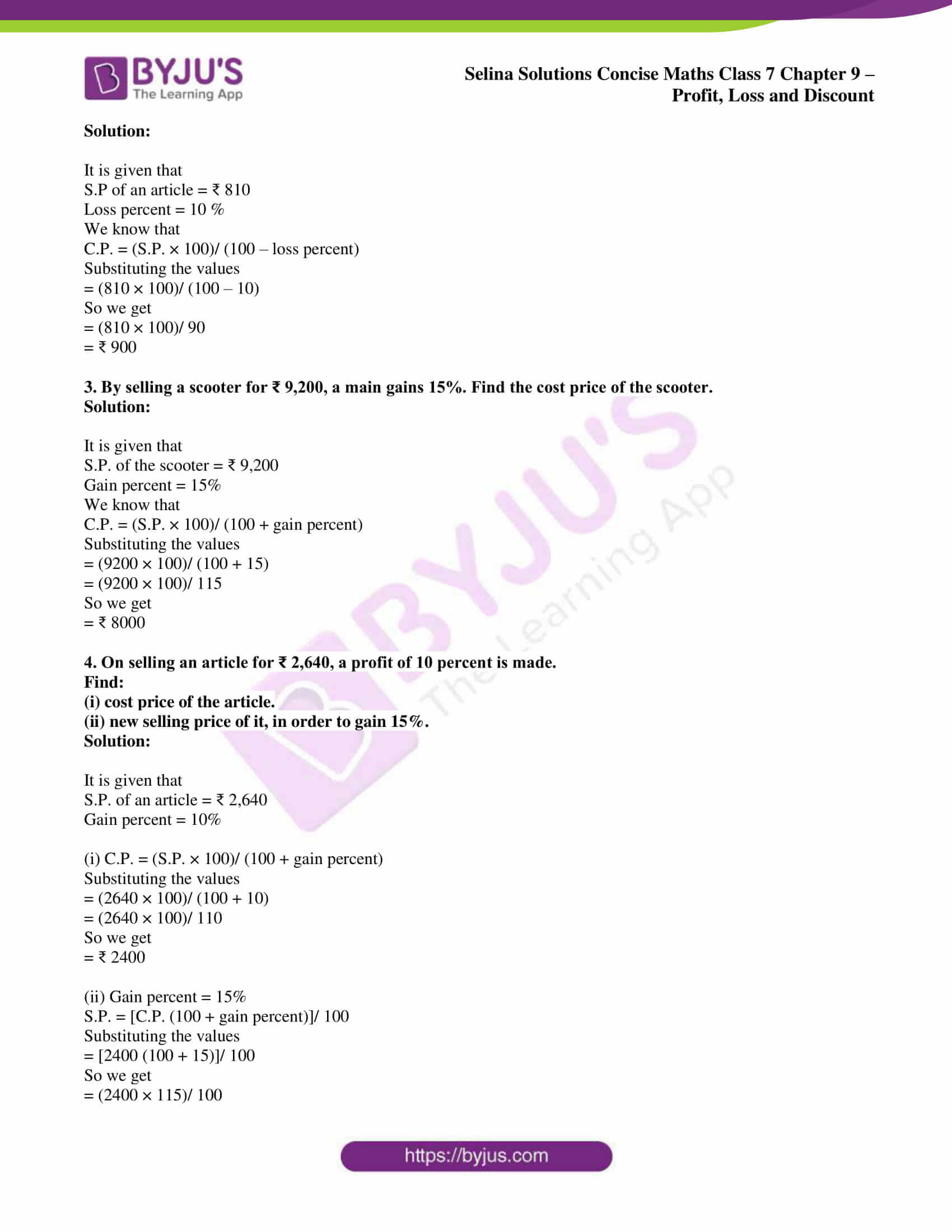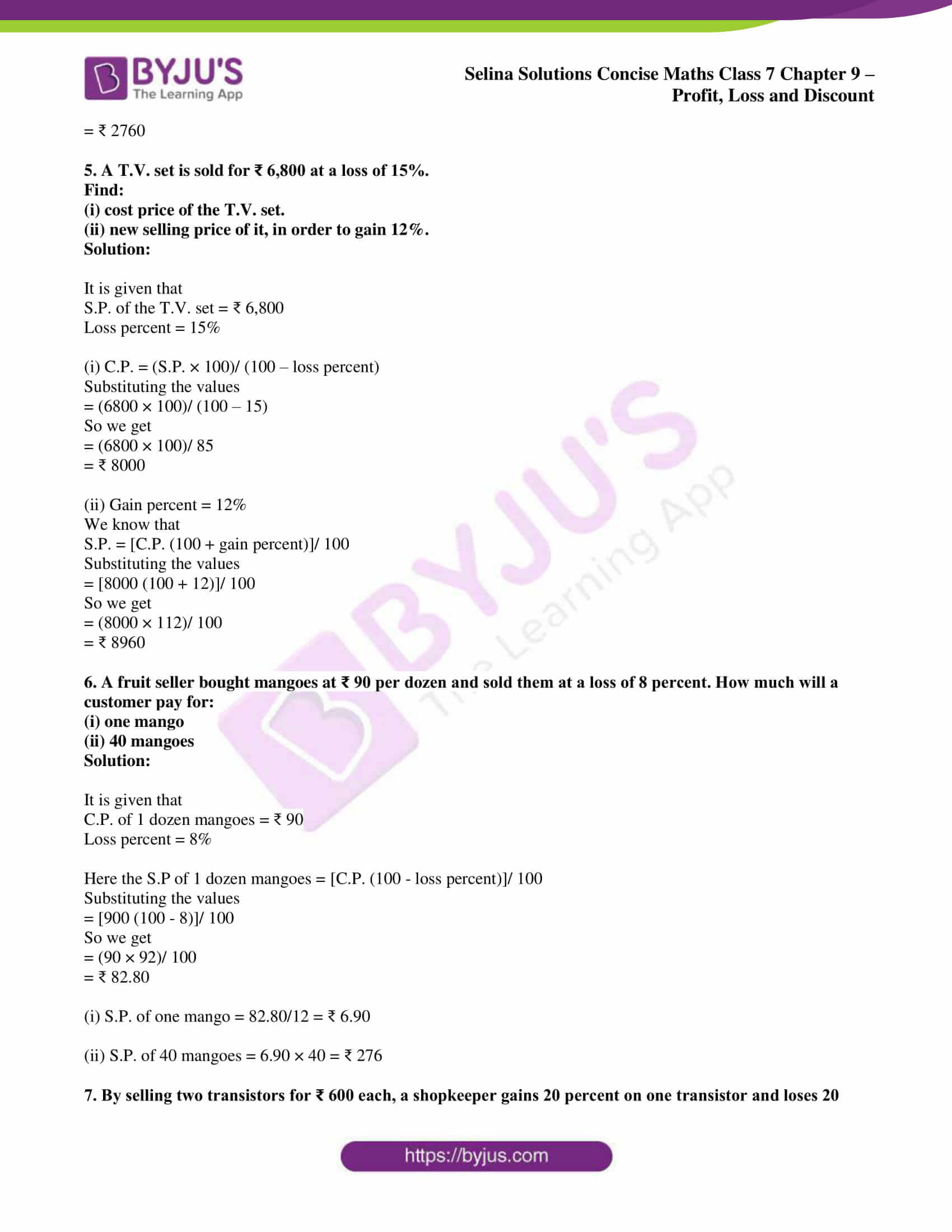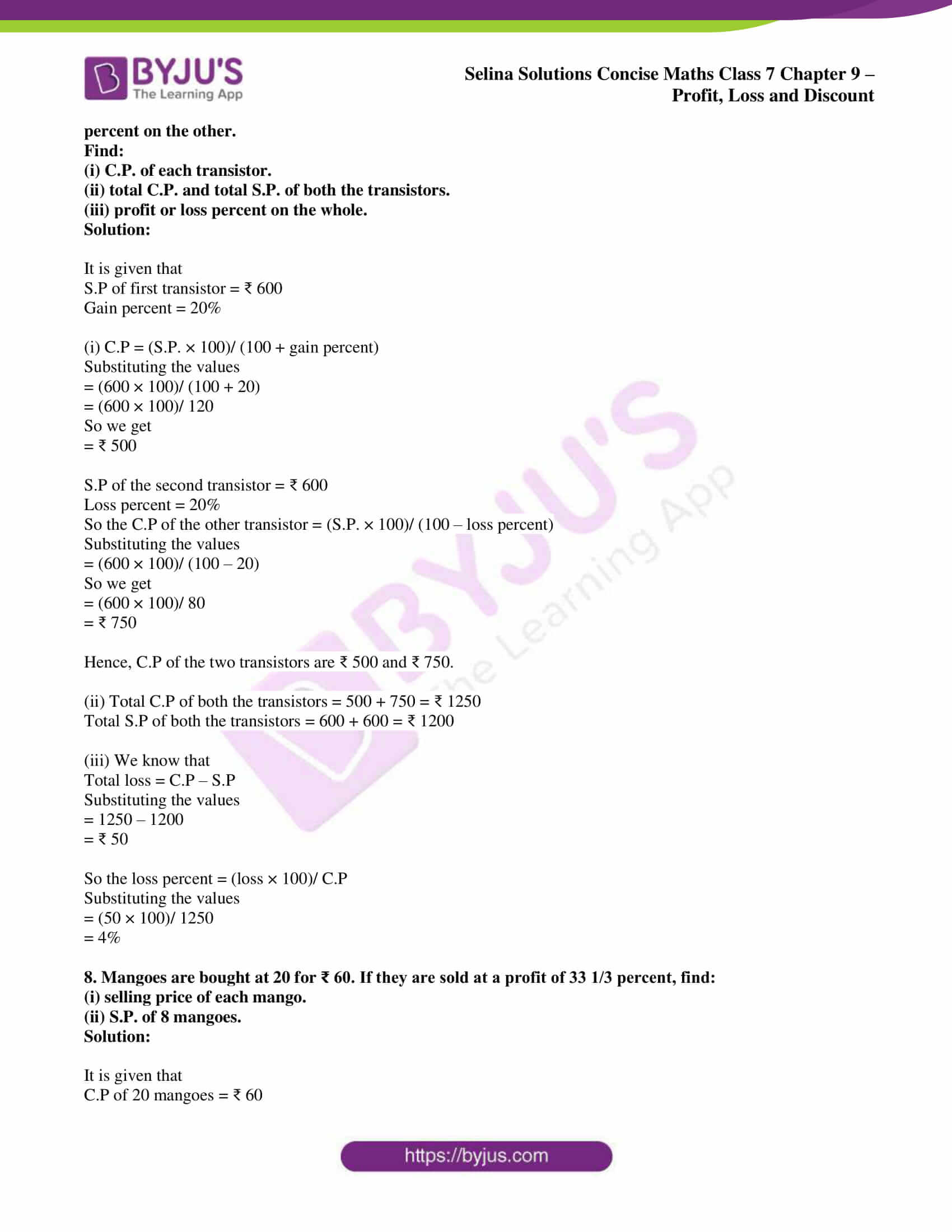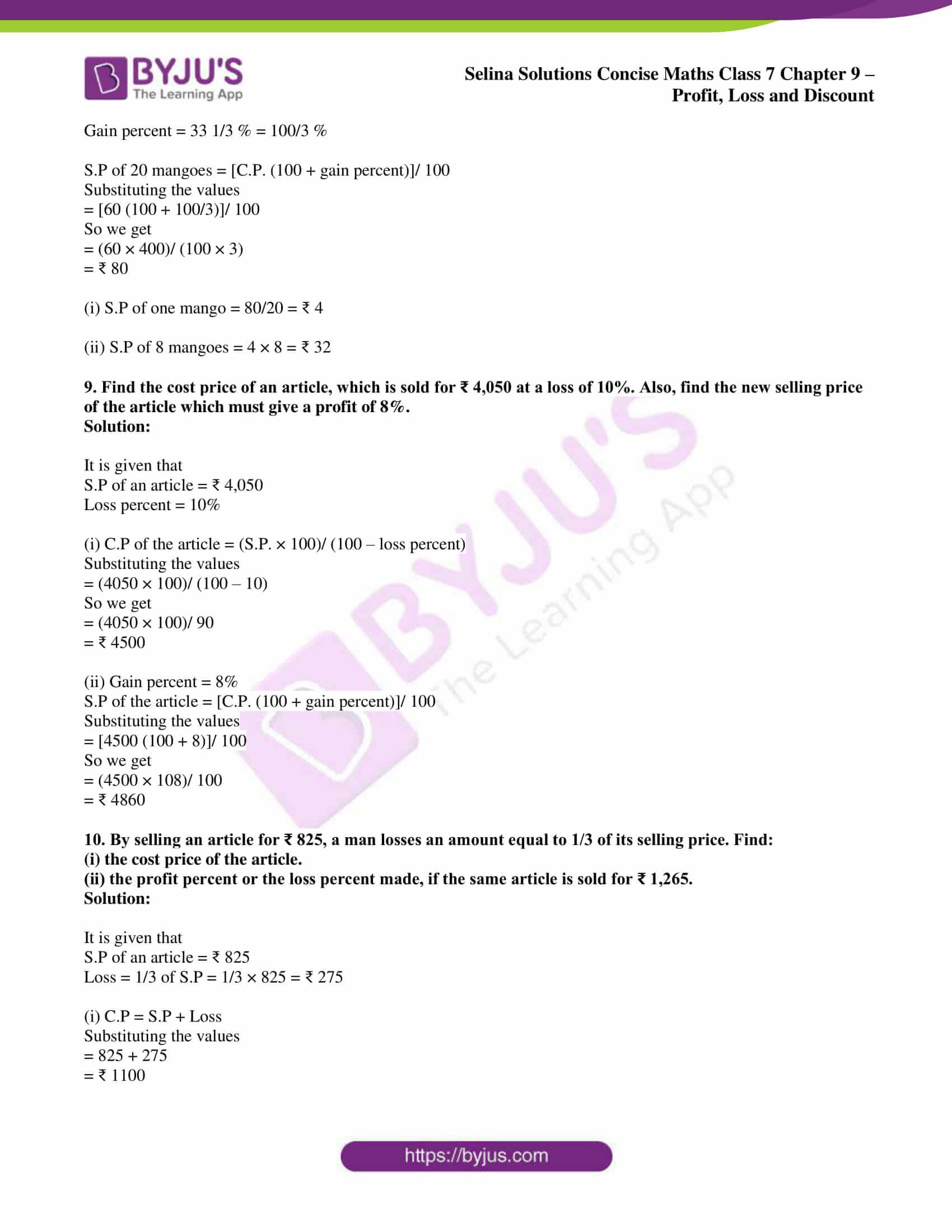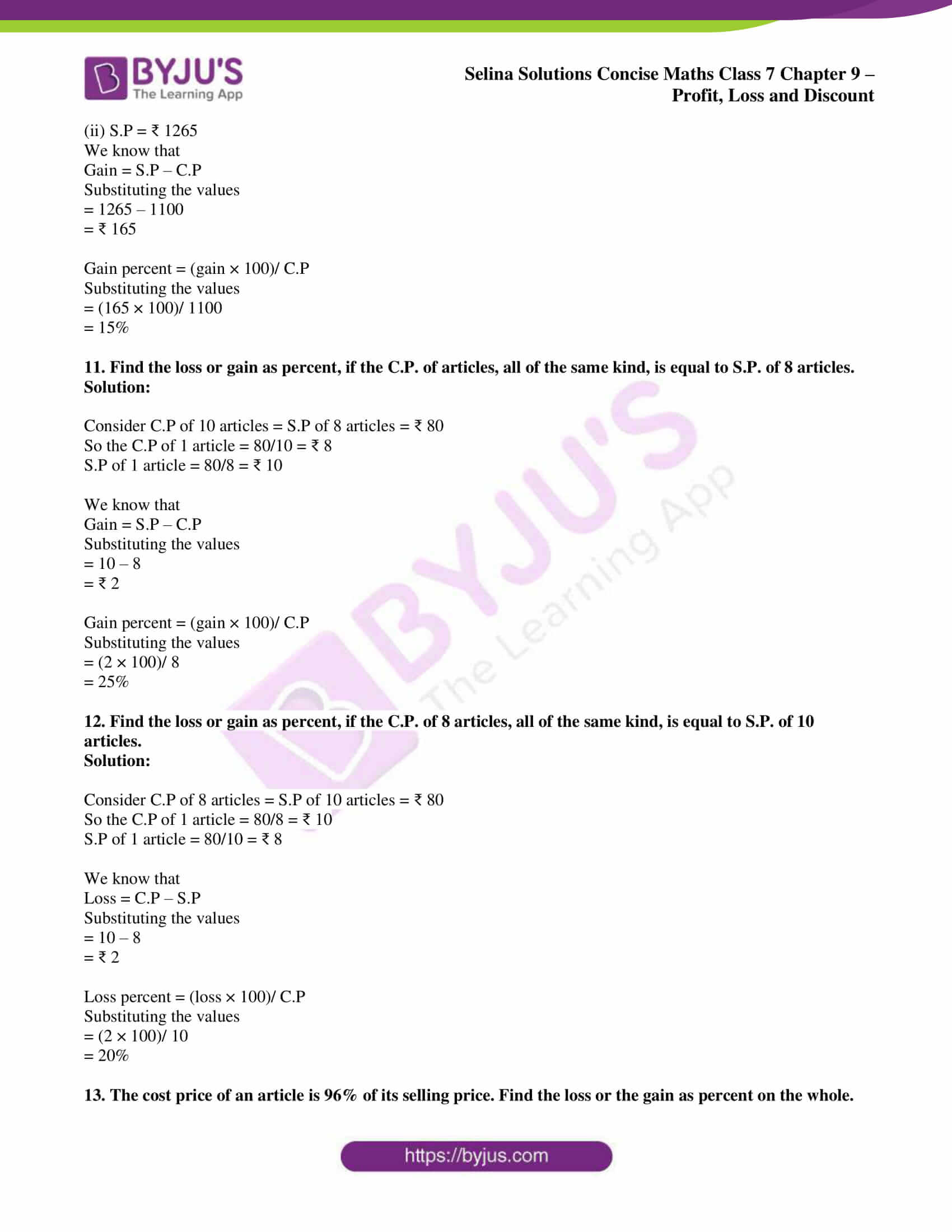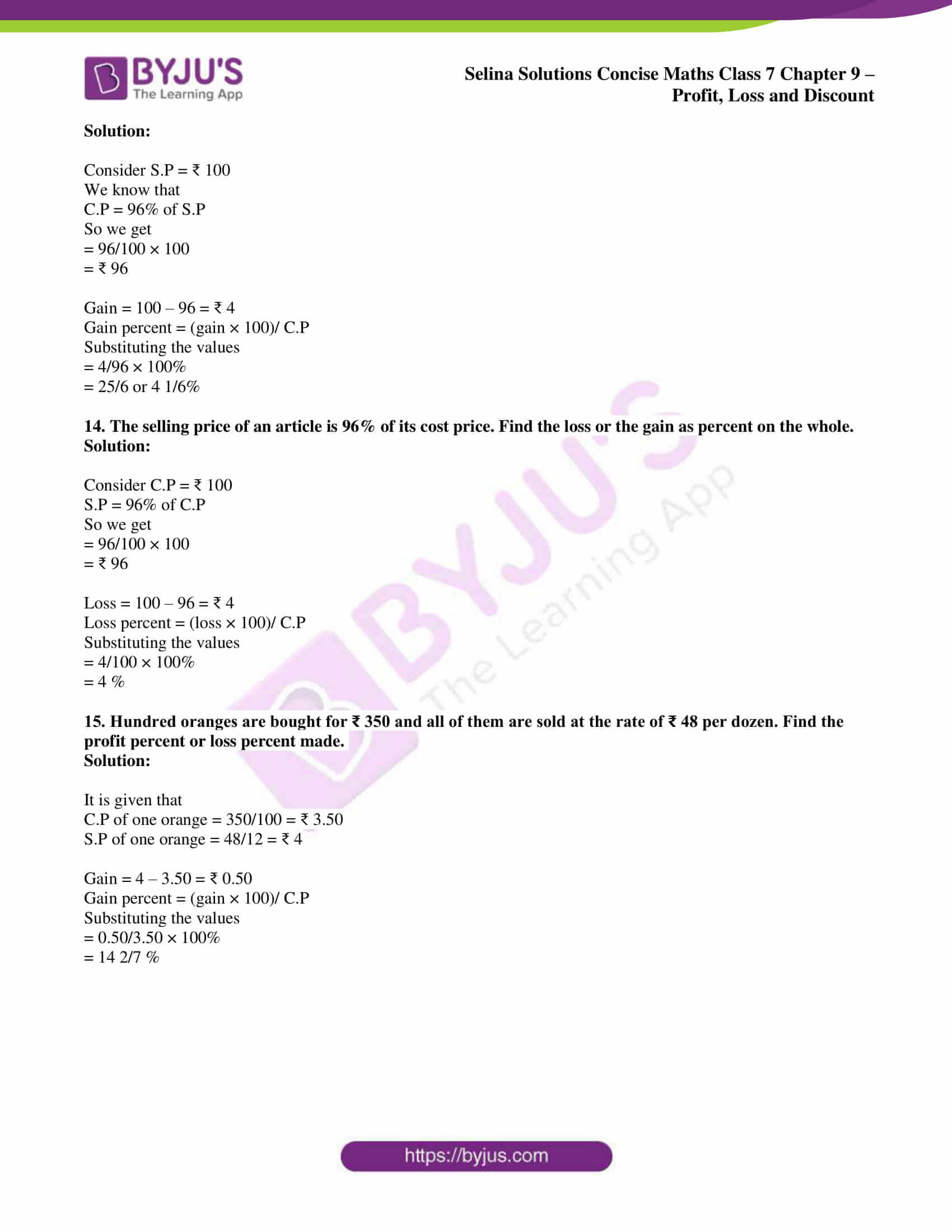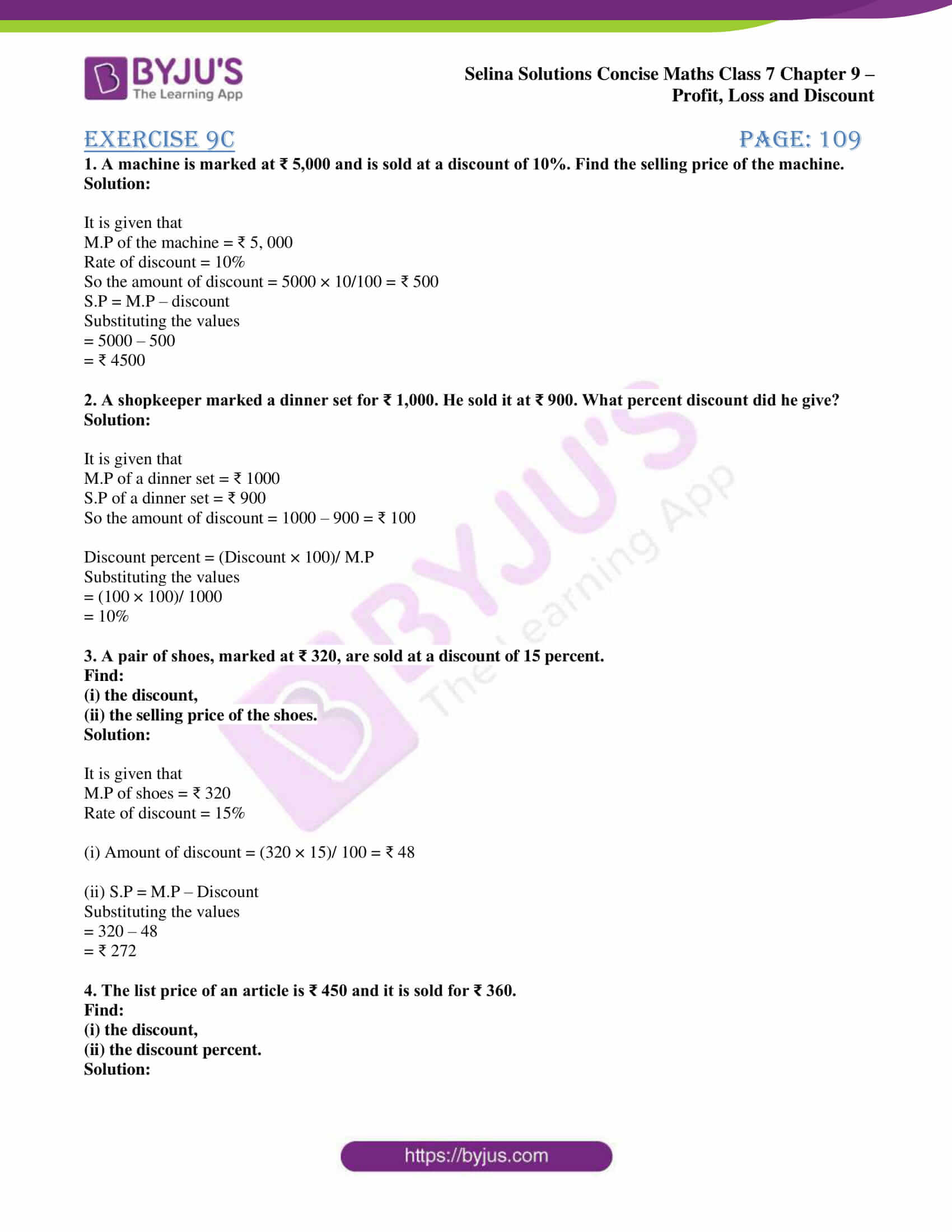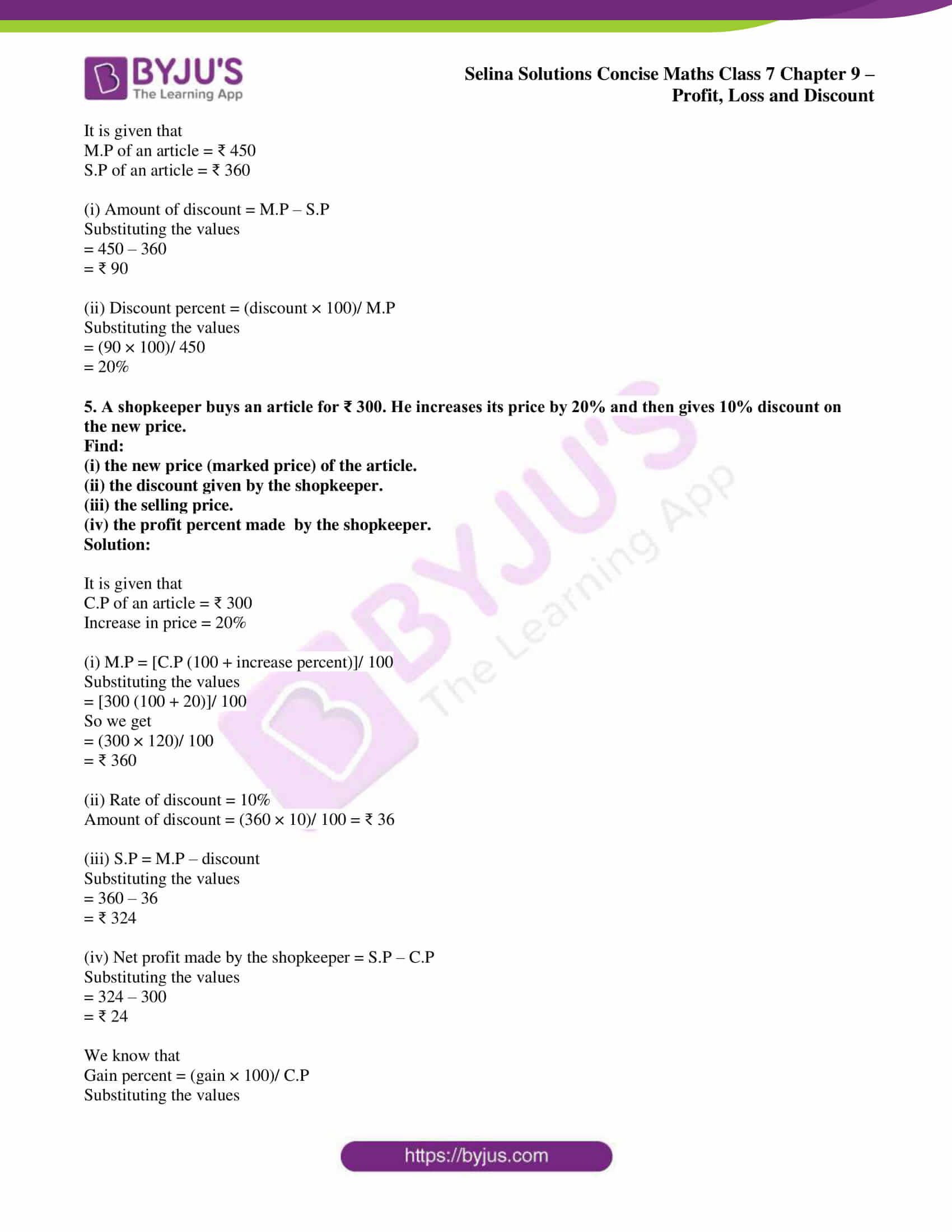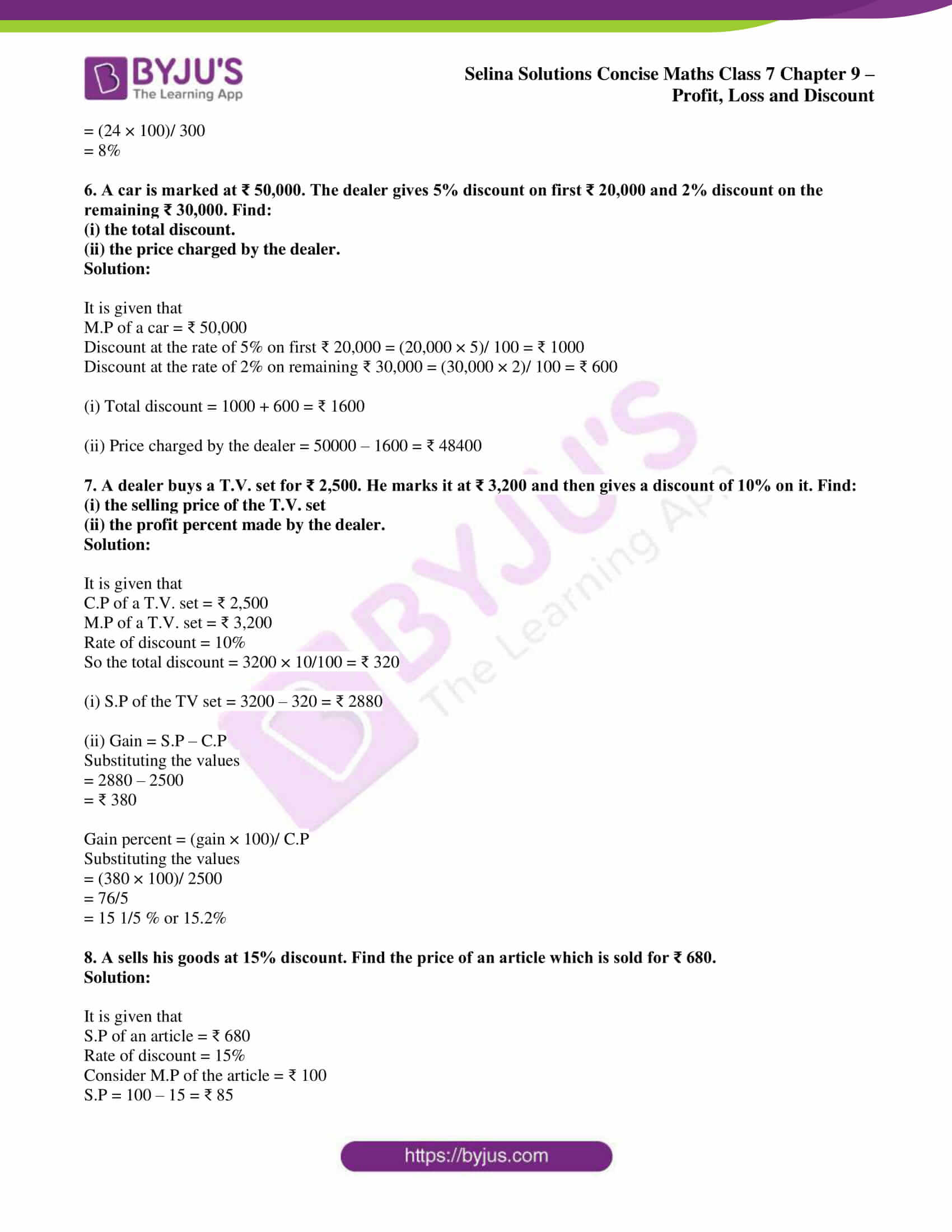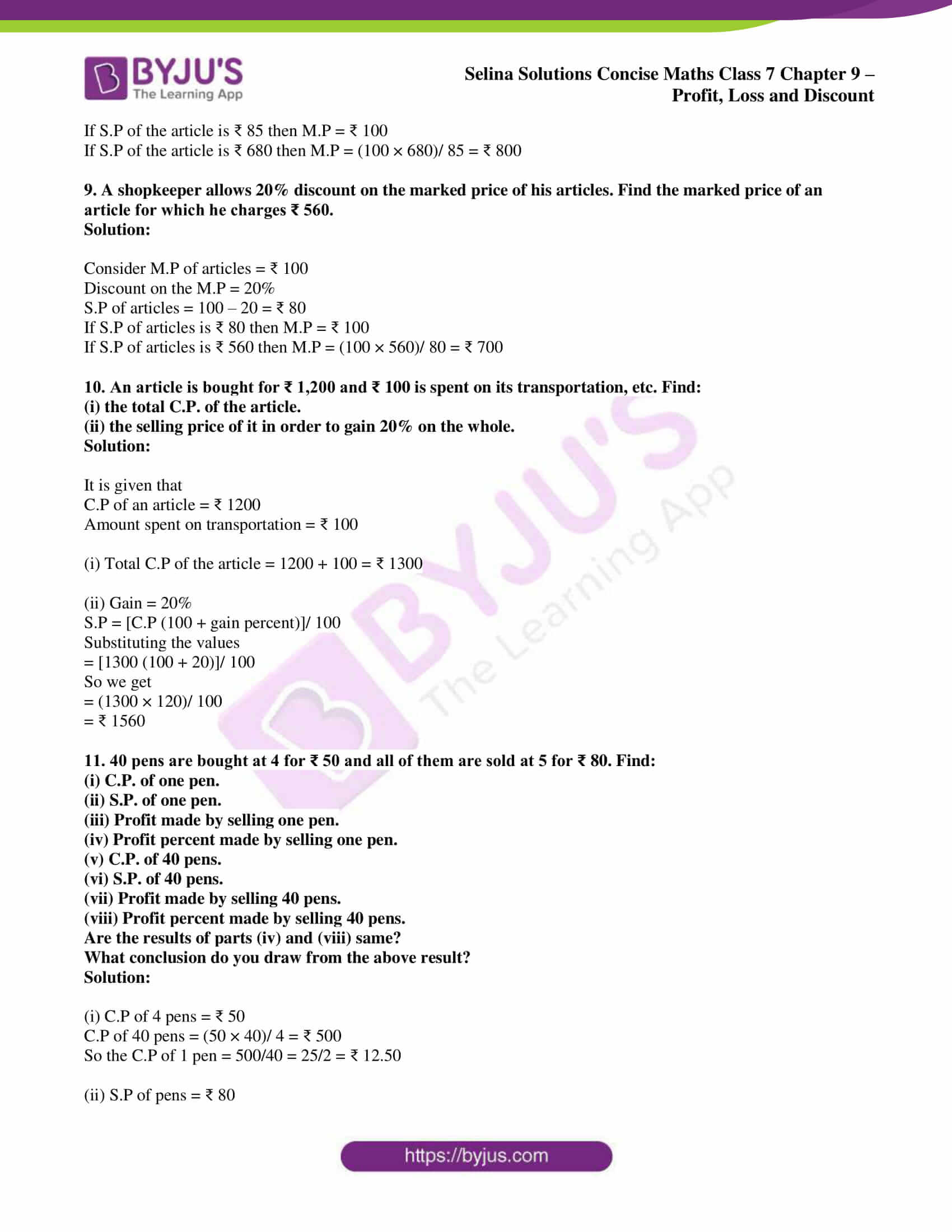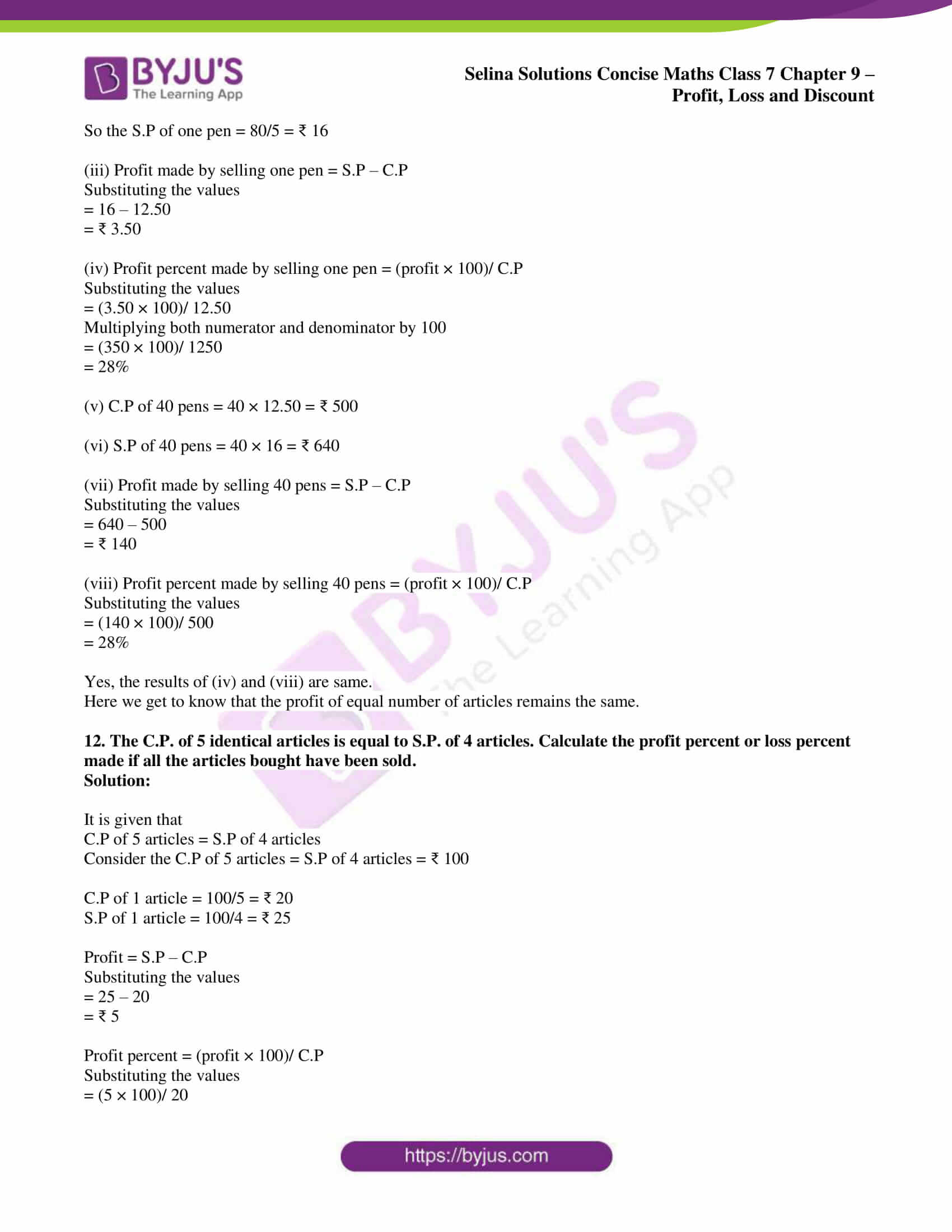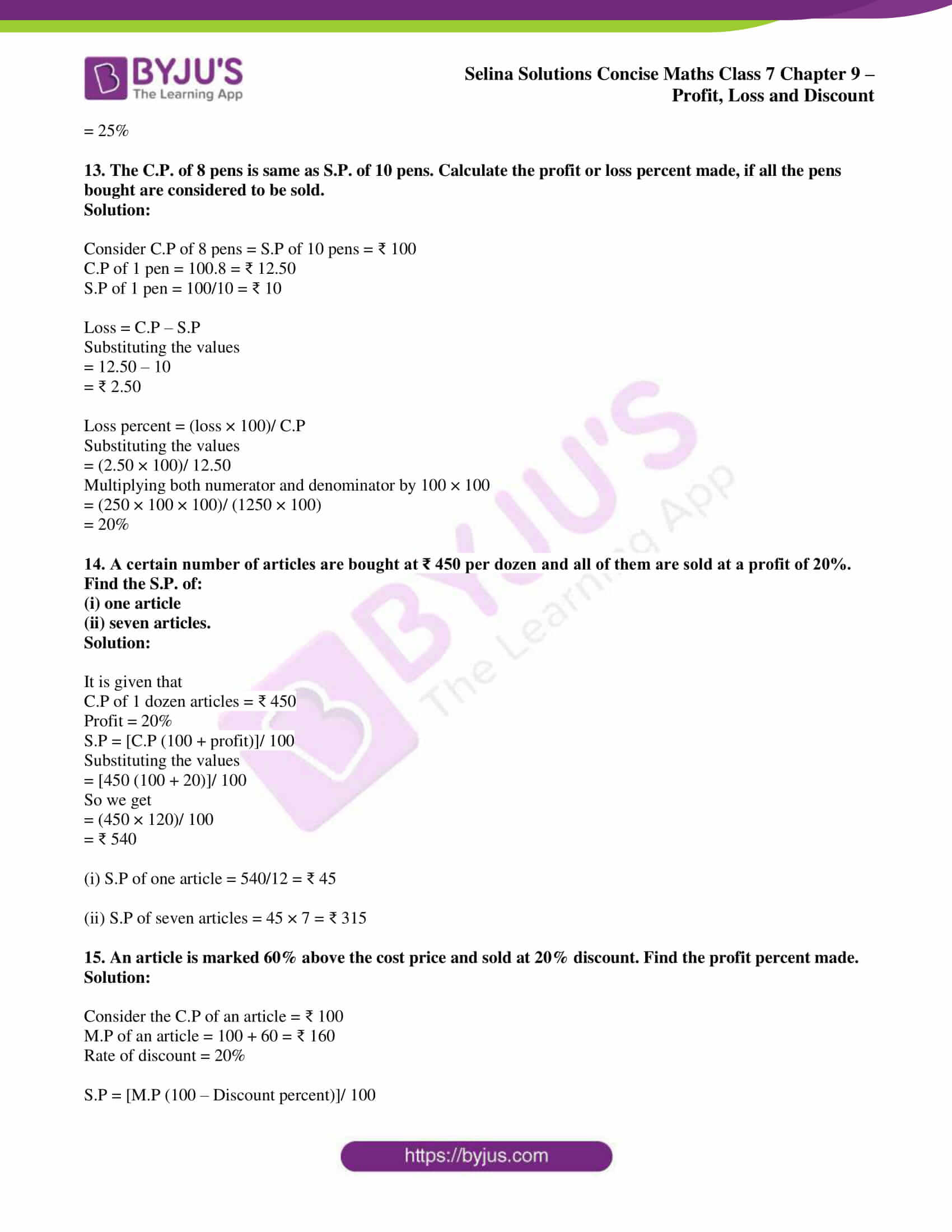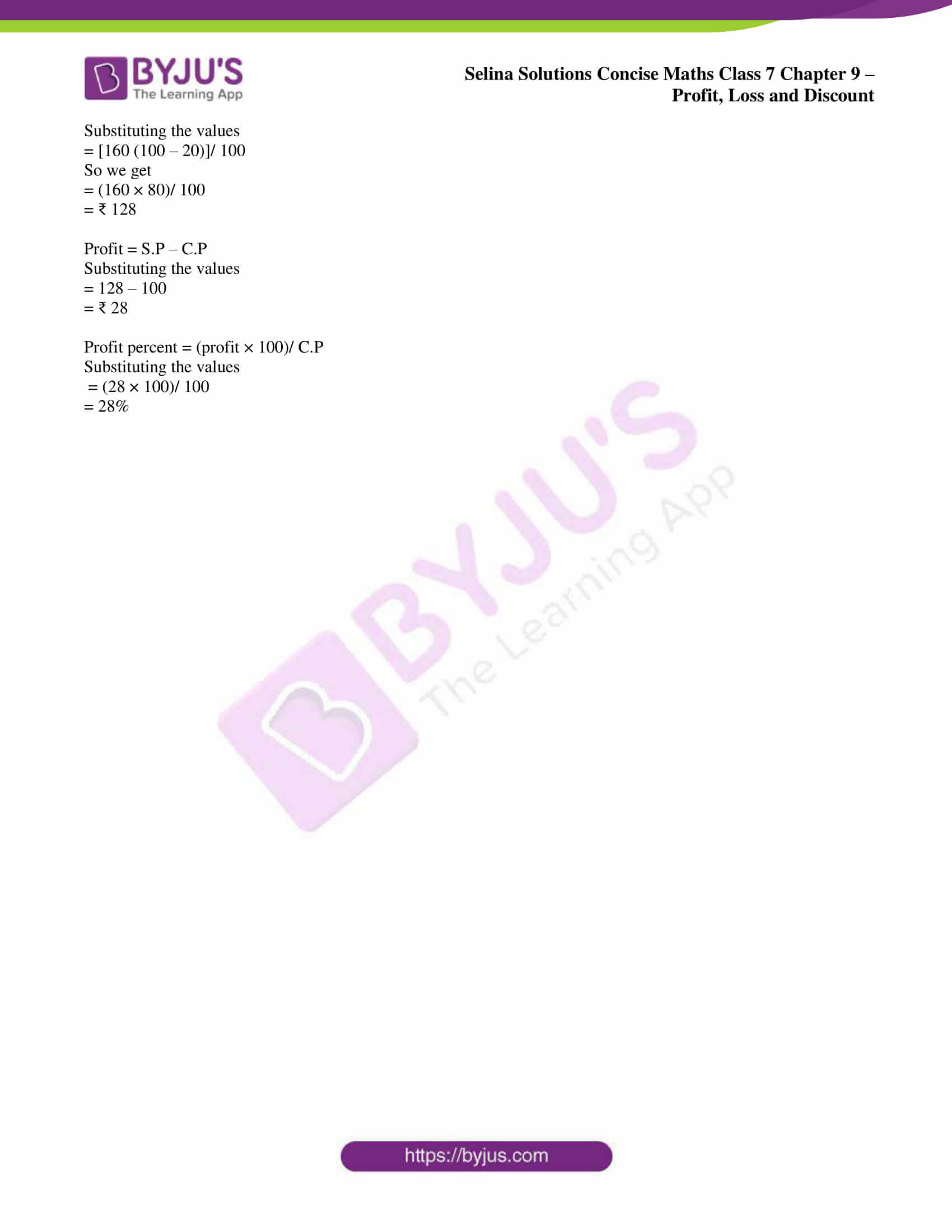## Access Selina Solutions Concise Maths Class 7 Chapter 9: Profit, Loss and Discount

#### Exercise 9A page: 104

1. Find the gain or loss percent, if:

(i) C.P. = ₹ 200 and S.P. = ₹ 224

(ii) C.P. = ₹ 450 and S.P. = ₹ 400

(iii) C.P. = ₹ 550 and gain = ₹ 22

(iv) C.P. = ₹ 216 and loss = ₹ 72

(v) S.P. = ₹ 500 and loss = ₹ 100

Solution:

(i) C.P. = ₹ 200 and S.P. = ₹ 224

We know that

Gain = S.P. – C.P.

So we get

= 224 – 200

= ₹ 24

So we get

Gain percent = (gain × 100)/ C.P.

Substituting the values

= (24 × 100)/ 200

= 12%

(ii) C.P. = ₹ 450 and S.P. = ₹ 400

We know that

Loss = C.P. – S.P.

So we get

= 450 – 400

= ₹ 50

So we get

Loss percent = (loss × 100)/ C.P.

Substituting the values

= (50 × 100)/ 450

= 100/9

= 11 1/9%

(iii) C.P. = ₹ 550 and gain = ₹ 22

We know that

S.P. = C.P. + gain

So we get

= 550 + 22

= ₹ 572

So we get

Gain percent = (gain × 200)/ C.P.

Substituting the values

= (22 × 100)/ 550

= 4%

(iv) C.P. = ₹ 216 and loss = ₹ 72

We know that

S.P. = C.P. – loss

So we get

= 216 – 72

= ₹ 144

So we get

Loss percent = (loss × 100)/ C.P.

Substituting the values

= (72 × 100)/ 216

= 100/3

= 33 1/3%

(v) S.P. = ₹ 500 and loss = ₹ 100

We know that

C.P. = S.P. + loss

So we get

= 500 + 100

= ₹ 600

So we get

Loss percent = (loss × 100)/ C.P.

Substituting the values

= (100 × 100)/ 600

= 50/3

= 16 2/3 %

2. Find the selling price, if:

(i) C.P = ₹ 500 and gain = 25%

(ii) C.P. = ₹ 60 and loss = 12 ½ %

Solution:

(i) C.P = ₹ 500 and gain = 25%

We know that

S.P. = [C.P. (100 + gain percent)]/ 100

Substituting the values

= [500 (100 + 25)]/ 100

We get

= (500 × 125)/ 100

= ₹ 625

(ii) C.P. = ₹ 60 and loss = 12 ½ %

We know that

Loss = 12 ½ % = 25/2%

Here

S.P. = [C.P. (100 – Loss percent)]/ 100

Substituting the values

= [60 (100 – 25/2)]/ 100

So we get

= [60 (200 – 25/ 2)]/ 100

We can write it as

= (60 × 175)/ (2 × 100)

= ₹ 105/2

= ₹ 52.50

3. Rohit bought a tape-recorder for ₹ 1,500 and sold it for ₹ 1,800. Calculate his profit or loss percent.

Solution:

It is given that

C.P of tape-recorder = ₹ 1,500

S.P of tape-recorder = ₹ 1,800

We know that

Gain = S.P – C. P

= 1800 – 1500

= ₹ 300

Gain percent = (Gain × 100)/ C.P

Substituting the values

= (300 × 100)/ 1500

= 20%

4. An article bought for ₹ 350 is sold at a profit of 20%. Find its selling price.

Solution:

It is given that

C.P of an article = ₹ 350

Profit = 20%

We know that

S.P = [C.P (100 + profit percent)]/ 100

Substituting the values

= [350 (100 + 20)]/ 100

So we get

= (350 × 120)/ 100

= ₹ 420

5. An old machine is bought for ₹ 1,400 and is sold at a loss of 15%. Find its selling price.

Solution:

It is given that

C.P. of the old machine = ₹ 1,400

Loss percent = 15%

We know that

S.P = [C.P (100 – loss percent)]/ 100

Substituting the values

= [1400 (100 – 15)]/ 100

By further calculation

= (1400 × 85)/ 100

= ₹ 1190

6. Oranges are bought at 5 for ₹ 10 and sold at 6 for ₹ 15. Find profit or loss as percent.

Solution:

We know that

LCM of 5 and 6 = 30

Consider that 30 oranges are bought

So the C.P of 30 oranges = (30 × 10)/ 5 = ₹ 60

S.P of 30 oranges = (30 × 15)/ 6 = ₹ 75

Gain = S.P – C.P

Substituting the values

= 75 – 60

= ₹ 15

Gain percent = (gain × 100)/ C.P

Substituting the values

= (15 × 100)/ 60

= 25%

7. A certain number of articles are bought at 3 for ₹ 150 and all of them are sold at 4 for ₹ 180. Find the loss or gain as percent.

Solution:

We know that

LCM of 3 and 4 = 12

Consider that 12 articles are bought

So the C.P of 12 articles = (150 × 12)/ 3 = ₹ 600

S.P of 12 articles = (180 × 12)/ 4 = ₹ 540

Loss = C.P – S.P

Substituting the values

= 600 – 540

= ₹ 60

Loss percent = (loss × 100)/ C.P

Substituting the values

= (60 × 100)/ 600

= 10%

8. A vendor bought 120 sweets at 20 p each. In his house, 18 were consumed and he sold the remaining at 30p each. Find his profit or loss as percent.

Solution:

No. of sweets bought = 120

C.P of 120 sweets = (120 × 20)/ 100 = ₹ 24

No. of sweets consumed = 18

So the balance sweets = 120 – 18 = 102

S.P of 102 sweets = (102 × 30)/ 100 = ₹ 30.60

Gain = S.P – C.P

Substituting the values

= 30.60 – 24

= ₹ 6.60

Gain percent = (gain × 100)/ C.P

Substituting the values

= (6.60 × 100)/ 24

Multiplying both numerator and denominator by 100

= (660 × 100)/ (100 × 24)

= 55/2

= 27.5%

9. The cost price of an article is ₹ 1,200 and selling price is 5/4 times of its cost price.

Find:

(i) Selling price of the article,

(ii) Profit or loss as percent.

Solution:

It is given that

C.P of an article = ₹ 1,200

We know that

S.P = 5/4 of C.P

Substituting the values

S.P = 5/4 × 1200 = ₹ 1,500

Gain = S.P – C.P

Substituting the values

= 1500 – 1200

= ₹ 300

Gain percent = (gain × 100)/ C.P

Substituting the values

= (300 × 100)/ 1200

= 25%

10. The selling price of an article is ₹ 1,200 and cost price is 5/4 times of its selling price.

Find:

(i) cost price of the article,

(ii) profit or loss as percent.

Solution:

(i) S.P of an article = ₹ 1,200

We know that

C.P = 5/4 of S.P

Substituting the values

= 5/4 × 1200

= ₹ 1,500

(ii) Loss = C.P – S.P

Substituting the values

= 1500 – 1200

= ₹ 300

Loss percent = (loss × 100)/ C.P

Substituting the values

= (300 × 100)/ 1500

So we get

= 100/5

= 20%

#### Exercise 9B page: 107

1. Find the cost price, if:

(i) S.P. = ₹ 21 and gain = 5%

(ii) S.P. = ₹ 22 and loss = 12%

(iii) S.P. = ₹ 340 and gain = ₹ 20

(iv) S.P. = ₹ 200 and loss = ₹ 50

(v) S.P. = ₹ 1 and loss = 5 p

Solution:

(i) S.P. = ₹ 21 and gain = 5%

We know that

C.P. = (S.P. × 100)/ (100 + gain percent)

Substituting the values

= (21 × 100)/ (100 + 5)

= (21 × 100)/ 105

So we get

= ₹ 20

(ii) S.P. = ₹ 22 and loss = 12%

We know that

C.P. = (S.P. × 100)/ (100 – loss percent)

Substituting the values

= (22 × 100)/ (100 – 12)

= (22 × 100)/ 88

So we get

= ₹ 25

(iii) S.P. = ₹ 340 and gain = ₹ 20

We know that

C.P. = S.P. – Gain

Substituting the values

= 340 – 20

= ₹ 320

(iv) S.P. = ₹ 200 and loss = ₹ 50

We know that

C.P. = S.P. + loss

Substituting the values

= 200 + 50

= ₹ 250

(v) S.P. = ₹ 1 and loss = 5 p

We know that

C.P. = S.P. + Loss

Substituting the values

= ₹ 1 + 5 p

= ₹ 1.05

2. By selling an article for ₹ 810, a loss of percent is suffered. Find its cost price.

Solution:

It is given that

S.P of an article = ₹ 810

Loss percent = 10 %

We know that

C.P. = (S.P. × 100)/ (100 – loss percent)

Substituting the values

= (810 × 100)/ (100 – 10)

So we get

= (810 × 100)/ 90

= ₹ 900

3. By selling a scooter for ₹ 9,200, a main gains 15%. Find the cost price of the scooter.

Solution:

It is given that

S.P. of the scooter = ₹ 9,200

Gain percent = 15%

We know that

C.P. = (S.P. × 100)/ (100 + gain percent)

Substituting the values

= (9200 × 100)/ (100 + 15)

= (9200 × 100)/ 115

So we get

= ₹ 8000

4. On selling an article for ₹ 2,640, a profit of 10 percent is made.

Find:

(i) cost price of the article.

(ii) new selling price of it, in order to gain 15%.

Solution:

It is given that

S.P. of an article = ₹ 2,640

Gain percent = 10%

(i) C.P. = (S.P. × 100)/ (100 + gain percent)

Substituting the values

= (2640 × 100)/ (100 + 10)

= (2640 × 100)/ 110

So we get

= ₹ 2400

(ii) Gain percent = 15%

S.P. = [C.P. (100 + gain percent)]/ 100

Substituting the values

= [2400 (100 + 15)]/ 100

So we get

= (2400 × 115)/ 100

= ₹ 2760

5. A T.V. set is sold for ₹ 6,800 at a loss of 15%.

Find:

(i) cost price of the T.V. set.

(ii) new selling price of it, in order to gain 12%.

Solution:

It is given that

S.P. of the T.V. set = ₹ 6,800

Loss percent = 15%

(i) C.P. = (S.P. × 100)/ (100 – loss percent)

Substituting the values

= (6800 × 100)/ (100 – 15)

So we get

= (6800 × 100)/ 85

= ₹ 8000

(ii) Gain percent = 12%

We know that

S.P. = [C.P. (100 + gain percent)]/ 100

Substituting the values

= [8000 (100 + 12)]/ 100

So we get

= (8000 × 112)/ 100

= ₹ 8960

6. A fruit seller bought mangoes at ₹ 90 per dozen and sold them at a loss of 8 percent. How much will a customer pay for:

(i) one mango

(ii) 40 mangoes

Solution:

It is given that

C.P. of 1 dozen mangoes = ₹ 90

Loss percent = 8%

Here the S.P of 1 dozen mangoes = [C.P. (100 – loss percent)]/ 100

Substituting the values

= [900 (100 – 8)]/ 100

So we get

= (90 × 92)/ 100

= ₹ 82.80

(i) S.P. of one mango = 82.80/12 = ₹ 6.90

(ii) S.P. of 40 mangoes = 6.90 × 40 = ₹ 276

7. By selling two transistors for ₹ 600 each, a shopkeeper gains 20 percent on one transistor and loses 20 percent on the other.

Find:

(i) C.P. of each transistor.

(ii) total C.P. and total S.P. of both the transistors.

(iii) profit or loss percent on the whole.

Solution:

It is given that

S.P of first transistor = ₹ 600

Gain percent = 20%

(i) C.P = (S.P. × 100)/ (100 + gain percent)

Substituting the values

= (600 × 100)/ (100 + 20)

= (600 × 100)/ 120

So we get

= ₹ 500

S.P of the second transistor = ₹ 600

Loss percent = 20%

So the C.P of the other transistor = (S.P. × 100)/ (100 – loss percent)

Substituting the values

= (600 × 100)/ (100 – 20)

So we get

= (600 × 100)/ 80

= ₹ 750

Hence, C.P of the two transistors are ₹ 500 and ₹ 750.

(ii) Total C.P of both the transistors = 500 + 750 = ₹ 1250

Total S.P of both the transistors = 600 + 600 = ₹ 1200

(iii) We know that

Total loss = C.P – S.P

Substituting the values

= 1250 – 1200

= ₹ 50

So the loss percent = (loss × 100)/ C.P

Substituting the values

= (50 × 100)/ 1250

= 4%

8. Mangoes are bought at 20 for ₹ 60. If they are sold at a profit of 33 1/3 percent, find:

(i) selling price of each mango.

(ii) S.P. of 8 mangoes.

Solution:

It is given that

C.P of 20 mangoes = ₹ 60

Gain percent = 33 1/3 % = 100/3 %

S.P of 20 mangoes = [C.P. (100 + gain percent)]/ 100

Substituting the values

= [60 (100 + 100/3)]/ 100

So we get

= (60 × 400)/ (100 × 3)

= ₹ 80

(i) S.P of one mango = 80/20 = ₹ 4

(ii) S.P of 8 mangoes = 4 × 8 = ₹ 32

9. Find the cost price of an article, which is sold for ₹ 4,050 at a loss of 10%. Also, find the new selling price of the article which must give a profit of 8%.

Solution:

It is given that

S.P of an article = ₹ 4,050

Loss percent = 10%

(i) C.P of the article = (S.P. × 100)/ (100 – loss percent)

Substituting the values

= (4050 × 100)/ (100 – 10)

So we get

= (4050 × 100)/ 90

= ₹ 4500

(ii) Gain percent = 8%

S.P of the article = [C.P. (100 + gain percent)]/ 100

Substituting the values

= [4500 (100 + 8)]/ 100

So we get

= (4500 × 108)/ 100

= ₹ 4860

10. By selling an article for ₹ 825, a man losses an amount equal to 1/3 of its selling price. Find:

(i) the cost price of the article.

(ii) the profit percent or the loss percent made, if the same article is sold for ₹ 1,265.

Solution:

It is given that

S.P of an article = ₹ 825

Loss = 1/3 of S.P = 1/3 × 825 = ₹ 275

(i) C.P = S.P + Loss

Substituting the values

= 825 + 275

= ₹ 1100

(ii) S.P = ₹ 1265

We know that

Gain = S.P – C.P

Substituting the values

= 1265 – 1100

= ₹ 165

Gain percent = (gain × 100)/ C.P

Substituting the values

= (165 × 100)/ 1100

= 15%

11. Find the loss or gain as percent, if the C.P. of articles, all of the same kind, is equal to S.P. of 8 articles.

Solution:

Consider C.P of 10 articles = S.P of 8 articles = ₹ 80

So the C.P of 1 article = 80/10 = ₹ 8

S.P of 1 article = 80/8 = ₹ 10

We know that

Gain = S.P – C.P

Substituting the values

= 10 – 8

= ₹ 2

Gain percent = (gain × 100)/ C.P

Substituting the values

= (2 × 100)/ 8

= 25%

12. Find the loss or gain as percent, if the C.P. of 8 articles, all of the same kind, is equal to S.P. of 10 articles.

Solution:

Consider C.P of 8 articles = S.P of 10 articles = ₹ 80

So the C.P of 1 article = 80/8 = ₹ 10

S.P of 1 article = 80/10 = ₹ 8

We know that

Loss = C.P – S.P

Substituting the values

= 10 – 8

= ₹ 2

Loss percent = (loss × 100)/ C.P

Substituting the values

= (2 × 100)/ 10

= 20%

13. The cost price of an article is 96% of its selling price. Find the loss or the gain as percent on the whole.

Solution:

Consider S.P = ₹ 100

We know that

C.P = 96% of S.P

So we get

= 96/100 × 100

= ₹ 96

Gain = 100 – 96 = ₹ 4

Gain percent = (gain × 100)/ C.P

Substituting the values

= 4/96 × 100%

= 25/6 or 4 1/6%

14. The selling price of an article is 96% of its cost price. Find the loss or the gain as percent on the whole.

Solution:

Consider C.P = ₹ 100

S.P = 96% of C.P

So we get

= 96/100 × 100

= ₹ 96

Loss = 100 – 96 = ₹ 4

Loss percent = (loss × 100)/ C.P

Substituting the values

= 4/100 × 100%

= 4 %

15. Hundred oranges are bought for ₹ 350 and all of them are sold at the rate of ₹ 48 per dozen. Find the profit percent or loss percent made.

Solution:

It is given that

C.P of one orange = 350/100 = ₹ 3.50

S.P of one orange = 48/12 = ₹ 4

Gain = 4 – 3.50 = ₹ 0.50

Gain percent = (gain × 100)/ C.P

Substituting the values

= 0.50/3.50 × 100%

= 14 2/7 %

#### Exercise 9C page: 109

1. A machine is marked at ₹ 5,000 and is sold at a discount of 10%. Find the selling price of the machine.

Solution:

It is given that

M.P of the machine = ₹ 5, 000

Rate of discount = 10%

So the amount of discount = 5000 × 10/100 = ₹ 500

S.P = M.P – discount

Substituting the values

= 5000 – 500

= ₹ 4500

2. A shopkeeper marked a dinner set for ₹ 1,000. He sold it at ₹ 900. What percent discount did he give?

Solution:

It is given that

M.P of a dinner set = ₹ 1000

S.P of a dinner set = ₹ 900

So the amount of discount = 1000 – 900 = ₹ 100

Discount percent = (Discount × 100)/ M.P

Substituting the values

= (100 × 100)/ 1000

= 10%

3. A pair of shoes, marked at ₹ 320, are sold at a discount of 15 percent.

Find:

(i) the discount,

(ii) the selling price of the shoes.

Solution:

It is given that

M.P of shoes = ₹ 320

Rate of discount = 15%

(i) Amount of discount = (320 × 15)/ 100 = ₹ 48

(ii) S.P = M.P – Discount

Substituting the values

= 320 – 48

= ₹ 272

4. The list price of an article is ₹ 450 and it is sold for ₹ 360.

Find:

(i) the discount,

(ii) the discount percent.

Solution:

It is given that

M.P of an article = ₹ 450

S.P of an article = ₹ 360

(i) Amount of discount = M.P – S.P

Substituting the values

= 450 – 360

= ₹ 90

(ii) Discount percent = (discount × 100)/ M.P

Substituting the values

= (90 × 100)/ 450

= 20%

5. A shopkeeper buys an article for ₹ 300. He increases its price by 20% and then gives 10% discount on the new price.

Find:

(i) the new price (marked price) of the article.

(ii) the discount given by the shopkeeper.

(iii) the selling price.

(iv) the profit percent made by the shopkeeper.

Solution:

It is given that

C.P of an article = ₹ 300

Increase in price = 20%

(i) M.P = [C.P (100 + increase percent)]/ 100

Substituting the values

= [300 (100 + 20)]/ 100

So we get

= (300 × 120)/ 100

= ₹ 360

(ii) Rate of discount = 10%

Amount of discount = (360 × 10)/ 100 = ₹ 36

(iii) S.P = M.P – discount

Substituting the values

= 360 – 36

= ₹ 324

(iv) Net profit made by the shopkeeper = S.P – C.P

Substituting the values

= 324 – 300

= ₹ 24

We know that

Gain percent = (gain × 100)/ C.P

Substituting the values

= (24 × 100)/ 300

= 8%

6. A car is marked at ₹ 50,000. The dealer gives 5% discount on first ₹ 20,000 and 2% discount on the remaining ₹ 30,000. Find:

(i) the total discount.

(ii) the price charged by the dealer.

Solution:

It is given that

M.P of a car = ₹ 50,000

Discount at the rate of 5% on first ₹ 20,000 = (20,000 × 5)/ 100 = ₹ 1000

Discount at the rate of 2% on remaining ₹ 30,000 = (30,000 × 2)/ 100 = ₹ 600

(i) Total discount = 1000 + 600 = ₹ 1600

(ii) Price charged by the dealer = 50000 – 1600 = ₹ 48400

7. A dealer buys a T.V. set for ₹ 2,500. He marks it at ₹ 3,200 and then gives a discount of 10% on it. Find:

(i) the selling price of the T.V. set

(ii) the profit percent made by the dealer.

Solution:

It is given that

C.P of a T.V. set = ₹ 2,500

M.P of a T.V. set = ₹ 3,200

Rate of discount = 10%

So the total discount = 3200 × 10/100 = ₹ 320

(i) S.P of the TV set = 3200 – 320 = ₹ 2880

(ii) Gain = S.P – C.P

Substituting the values

= 2880 – 2500

= ₹ 380

Gain percent = (gain × 100)/ C.P

Substituting the values

= (380 × 100)/ 2500

= 76/5

= 15 1/5 % or 15.2%

8. A sells his goods at 15% discount. Find the price of an article which is sold for ₹ 680.

Solution:

It is given that

S.P of an article = ₹ 680

Rate of discount = 15%

Consider M.P of the article = ₹ 100

S.P = 100 – 15 = ₹ 85

If S.P of the article is ₹ 85 then M.P = ₹ 100

If S.P of the article is ₹ 680 then M.P = (100 × 680)/ 85 = ₹ 800

9. A shopkeeper allows 20% discount on the marked price of his articles. Find the marked price of an article for which he charges ₹ 560.

Solution:

Consider M.P of articles = ₹ 100

Discount on the M.P = 20%

S.P of articles = 100 – 20 = ₹ 80

If S.P of articles is ₹ 80 then M.P = ₹ 100

If S.P of articles is ₹ 560 then M.P = (100 × 560)/ 80 = ₹ 700

10. An article is bought for ₹ 1,200 and ₹ 100 is spent on its transportation, etc. Find:

(i) the total C.P. of the article.

(ii) the selling price of it in order to gain 20% on the whole.

Solution:

It is given that

C.P of an article = ₹ 1200

Amount spent on transportation = ₹ 100

(i) Total C.P of the article = 1200 + 100 = ₹ 1300

(ii) Gain = 20%

S.P = [C.P (100 + gain percent)]/ 100

Substituting the values

= [1300 (100 + 20)]/ 100

So we get

= (1300 × 120)/ 100

= ₹ 1560

11. 40 pens are bought at 4 for ₹ 50 and all of them are sold at 5 for ₹ 80. Find:

(i) C.P. of one pen.

(ii) S.P. of one pen.

(iii) Profit made by selling one pen.

(iv) Profit percent made by selling one pen.

(v) C.P. of 40 pens.

(vi) S.P. of 40 pens.

(vii) Profit made by selling 40 pens.

(viii) Profit percent made by selling 40 pens.

Are the results of parts (iv) and (viii) same?

What conclusion do you draw from the above result?

Solution:

(i) C.P of 4 pens = ₹ 50

C.P of 40 pens = (50 × 40)/ 4 = ₹ 500

So the C.P of 1 pen = 500/40 = 25/2 = ₹ 12.50

(ii) S.P of pens = ₹ 80

So the S.P of one pen = 80/5 = ₹ 16

(iii) Profit made by selling one pen = S.P – C.P

Substituting the values

= 16 – 12.50

= ₹ 3.50

(iv) Profit percent made by selling one pen = (profit × 100)/ C.P

Substituting the values

= (3.50 × 100)/ 12.50

Multiplying both numerator and denominator by 100

= (350 × 100)/ 1250

= 28%

(v) C.P of 40 pens = 40 × 12.50 = ₹ 500

(vi) S.P of 40 pens = 40 × 16 = ₹ 640

(vii) Profit made by selling 40 pens = S.P – C.P

Substituting the values

= 640 – 500

= ₹ 140

(viii) Profit percent made by selling 40 pens = (profit × 100)/ C.P

Substituting the values

= (140 × 100)/ 500

= 28%

Yes, the results of (iv) and (viii) are same.

Here we get to know that the profit of equal number of articles remains the same.

12. The C.P. of 5 identical articles is equal to S.P. of 4 articles. Calculate the profit percent or loss percent made if all the articles bought have been sold.

Solution:

It is given that

C.P of 5 articles = S.P of 4 articles

Consider the C.P of 5 articles = S.P of 4 articles = ₹ 100

C.P of 1 article = 100/5 = ₹ 20

S.P of 1 article = 100/4 = ₹ 25

Profit = S.P – C.P

Substituting the values

= 25 – 20

= ₹ 5

Profit percent = (profit × 100)/ C.P

Substituting the values

= (5 × 100)/ 20

= 25%

13. The C.P. of 8 pens is same as S.P. of 10 pens. Calculate the profit or loss percent made, if all the pens bought are considered to be sold.

Solution:

Consider C.P of 8 pens = S.P of 10 pens = ₹ 100

C.P of 1 pen = 100.8 = ₹ 12.50

S.P of 1 pen = 100/10 = ₹ 10

Loss = C.P – S.P

Substituting the values

= 12.50 – 10

= ₹ 2.50

Loss percent = (loss × 100)/ C.P

Substituting the values

= (2.50 × 100)/ 12.50

Multiplying both numerator and denominator by 100 × 100

= (250 × 100 × 100)/ (1250 × 100)

= 20%

14. A certain number of articles are bought at ₹ 450 per dozen and all of them are sold at a profit of 20%. Find the S.P. of:

(i) one article

(ii) seven articles.

Solution:

It is given that

C.P of 1 dozen articles = ₹ 450

Profit = 20%

S.P = [C.P (100 + profit)]/ 100

Substituting the values

= [450 (100 + 20)]/ 100

So we get

= (450 × 120)/ 100

= ₹ 540

(i) S.P of one article = 540/12 = ₹ 45

(ii) S.P of seven articles = 45 × 7 = ₹ 315

15. An article is marked 60% above the cost price and sold at 20% discount. Find the profit percent made.

Solution:

Consider the C.P of an article = ₹ 100

M.P of an article = 100 + 60 = ₹ 160

Rate of discount = 20%

S.P = [M.P (100 – Discount percent)]/ 100

Substituting the values

= [160 (100 – 20)]/ 100

So we get

= (160 × 80)/ 100

= ₹ 128

Profit = S.P – C.P

Substituting the values

= 128 – 100

= ₹ 28

Profit percent = (profit × 100)/ C.P

Substituting the values

= (28 × 100)/ 100

= 28%